# Welcome to GMCs Mathematics Brushup Packet for the

• Slides: 30
Download presentationWelcome to GMC’s Mathematics Brush-up Packet for the COMPASS Placement Exam!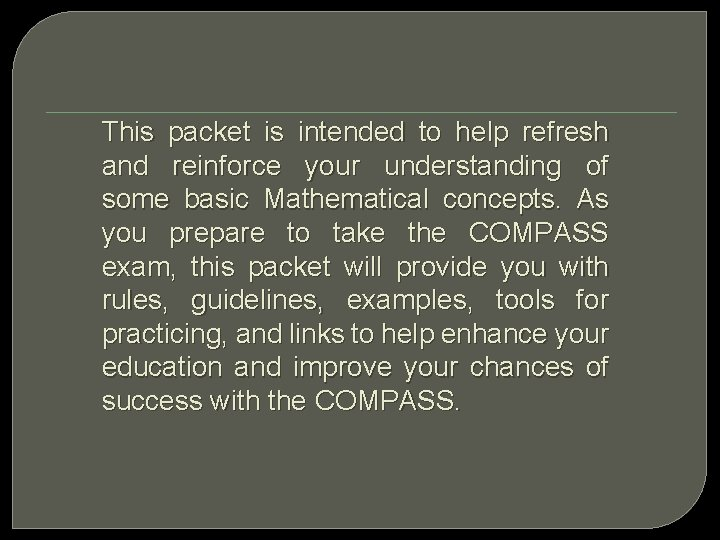This packet is intended to help refresh and reinforce your understanding of some basic Mathematical concepts. As you prepare to take the COMPASS exam, this packet will provide you with rules, guidelines, examples, tools for practicing, and links to help enhance your education and improve your chances of success with the COMPASS.Pre-Algebra SKILLS An understanding of these 8 skill sets will help you ace the COMPASS: üIntegers üDecimals üExponents üSquare roots üScientific notation üFractions üPercentages üAverages (means, medians, and modes)Algebra SKILLS An understanding of these 10 skill sets will help you ace the COMPASS: üSubstituting Values üSetting up equations üFactoring polynomials üExponents and radicals üBasic operations/polynomials üLinear equations/one variable üLinear equations/ two variables üRational expressions üQuadratic formulas üFunctionsIntegers �What are Integers? �Integers are the set of whole numbers and their opposites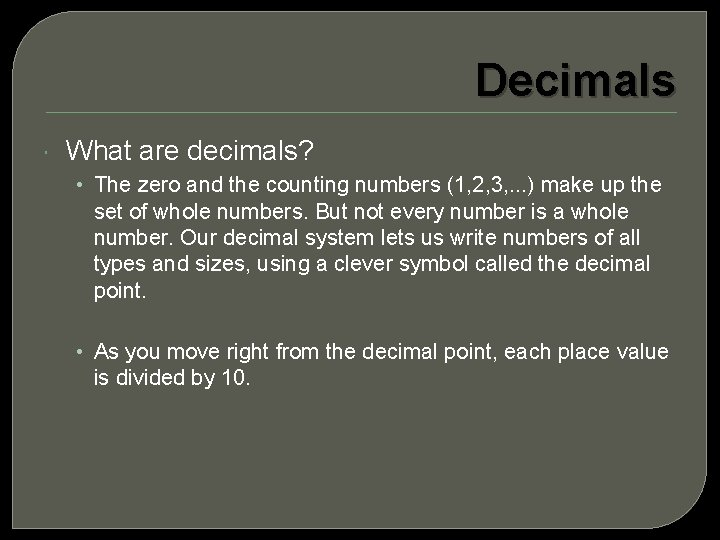Decimals What are decimals? • The zero and the counting numbers (1, 2, 3, . . . ) make up the set of whole numbers. But not every number is a whole number. Our decimal system lets us write numbers of all types and sizes, using a clever symbol called the decimal point. • As you move right from the decimal point, each place value is divided by 10.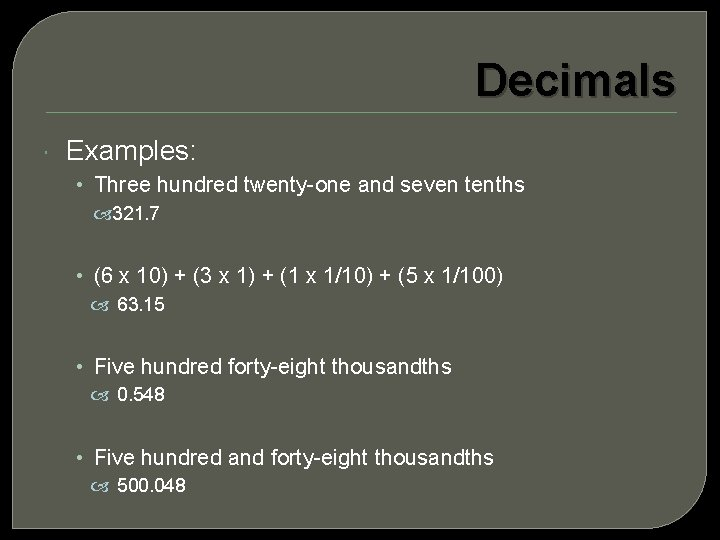Decimals Examples: • Three hundred twenty-one and seven tenths 321. 7 • (6 x 10) + (3 x 1) + (1 x 1/10) + (5 x 1/100) 63. 15 • Five hundred forty-eight thousandths 0. 548 • Five hundred and forty-eight thousandths 500. 048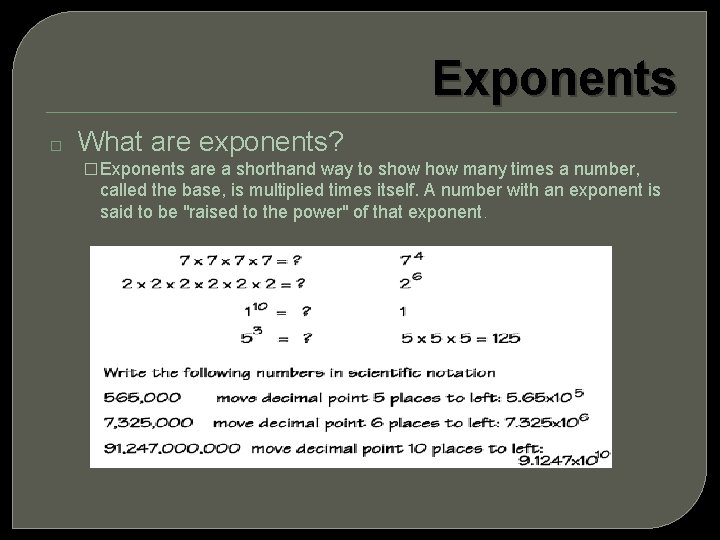Exponents � What are exponents? � Exponents are a shorthand way to show many times a number, called the base, is multiplied times itself. A number with an exponent is said to be "raised to the power" of that exponent.Square Roots Finding the square root of a number is the inverse operation of squaring that number. Remember, the square of a number is that number times itself. The perfect squares are the squares of the whole numbers. The square root of a number, n, written below is the number that gives n when multiplied by itself.Scientific Notations By using exponents, we can reformat numbers. For very large or very small numbers, it is sometimes simpler to use "scientific notation" (so called, because scientists often deal with very large and very small numbers). The format for writing a number in scientific notation is fairly simple: (first digit of the number) followed by (the decimal point) and then (all the rest of the digits of the number), times (10 to an appropriate power). The conversion is fairly simple. • Write 124 in scientific notation. • This is not a very large number, but it will work nicely for an example. To convert this to scientific notation, I first write "1. 24". This is not the same number, but (1. 24)(100) = 124 is, and 100 = 102. Then, in scientific notation, 124 is written as 1. 24 × 102.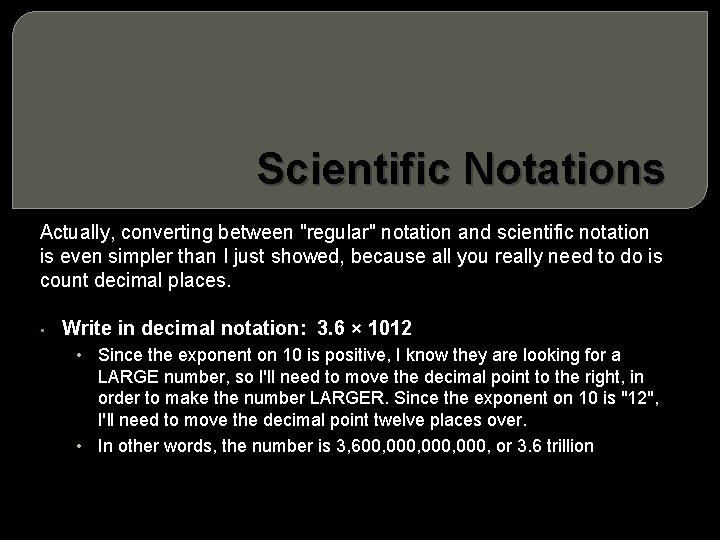Scientific Notations Actually, converting between "regular" notation and scientific notation is even simpler than I just showed, because all you really need to do is count decimal places. • Write in decimal notation: 3. 6 × 1012 • Since the exponent on 10 is positive, I know they are looking for a LARGE number, so I'll need to move the decimal point to the right, in order to make the number LARGER. Since the exponent on 10 is "12", I'll need to move the decimal point twelve places over. • In other words, the number is 3, 600, 000, or 3. 6 trillionFractions Adding and Subtracting Fractions: • Like fractions are fractions with the same denominator. You can add and subtract like fractions easily - simply add or subtract the numerators and write the sum over the common denominator. • Before you can add or subtract fractions with different denominators, you must first find equivalent fractions with the same denominator, like this: • Find the smallest multiple (LCM) of both numbers. • • When working with fractions, the LCM is called the least common denominator (LCD). Rewrite the fractions as equivalent fractions with the LCM as the denominator.Fractions Method 1: Write the multiples of both denominators until you find a common multiple. The first method is to simply start writing all the multiples of both denominators, beginning with the numbers themselves. Here's an example of this method. Multiples of 4 are 4, 8, 12, 16, and so forth (because 1 × 4=4, 2 × 4=8, 3 × 4=12, 4 × 4=16, etc. ). The multiples of 6 are 6, 12, …--that's the number we're looking for, 12, because it's the first one that appears in both lists of multiples. It's the least common multiple, which we'll use as our least common denominator.Fractions Method 1: Write the multiples of both denominators until you find a common multiple. The first method is to simply start writing all the multiples of both denominators, beginning with the numbers themselves. Here's an example of this method. Multiples of 4 are 4, 8, 12, 16, and so forth (because 1 × 4=4, 2 × 4=8, 3 × 4=12, 4 × 4=16, etc. ). The multiples of 6 are 6, 12, …--that's the number we're looking for, 12, because it's the first one that appears in both lists of multiples. It's the least common multiple, which we'll use as our least common denominator.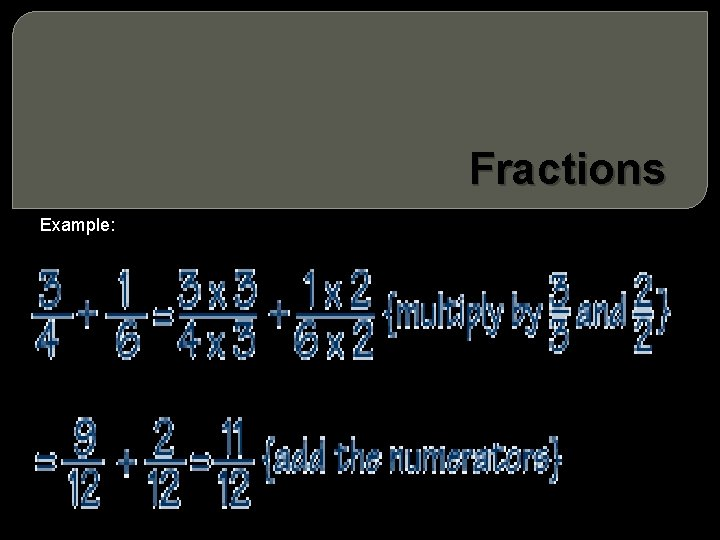Fractions Example:Fractions To multiply fractions: Simplify the fractions if not in lowest terms. Multiply the numerators of the fractions to get the new numerator. Multiply the denominators of the fractions to get the new denominator. Simplify the resulting fraction if possible.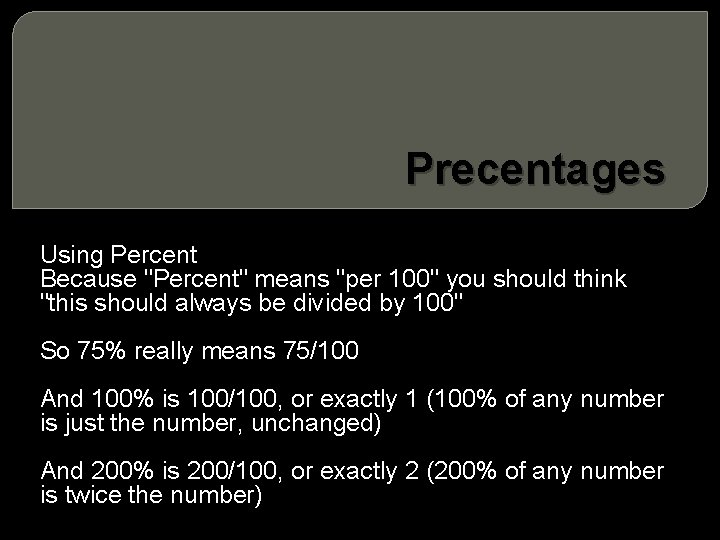Precentages Using Percent Because "Percent" means "per 100" you should think "this should always be divided by 100" So 75% really means 75/100 And 100% is 100/100, or exactly 1 (100% of any number is just the number, unchanged) And 200% is 200/100, or exactly 2 (200% of any number is twice the number)Averages (means, medians, and modes) • Mean • There are three main types of average: mean, mode and median. The mean is what most people mean when they say 'average'. It is found by adding up all of the numbers you have to find the mean of, and dividing by the number of numbers. • So the mean of 3, 5, 7, 3 and 5 is 23/5 = 4. 6.Averages (means, medians, and modes) Moving Averages A moving average is used to compare a set of figures over time. For example, suppose you have measured the weight of a child over an eight year period and have the following figures (in kg): 32, 33 , 35, 38, 43, 53, 63 , 65 Taking the mean doesn't give us much useful information. However, we could take the average of each 3 year period. These are the 3 -year moving averages. The first is: (32 + 33 + 35)/3 = 33. 3 The second is: (33 + 35 + 38)/3 = 35. 3 The third is: (35 + 38 + 43)/3 = 38. 7, and so on (there are 3 more!). To calculate the 4 year moving averages, you'd do 4 years at a time instead, and so on. . .Averages (means, medians, and modes) Mode The mode is the number in a set of numbers which occurs the most. So the modal value of 5, 6, 3, 4, 5, 2, 5 and 3 is 5, because there are more 5 s than any other number.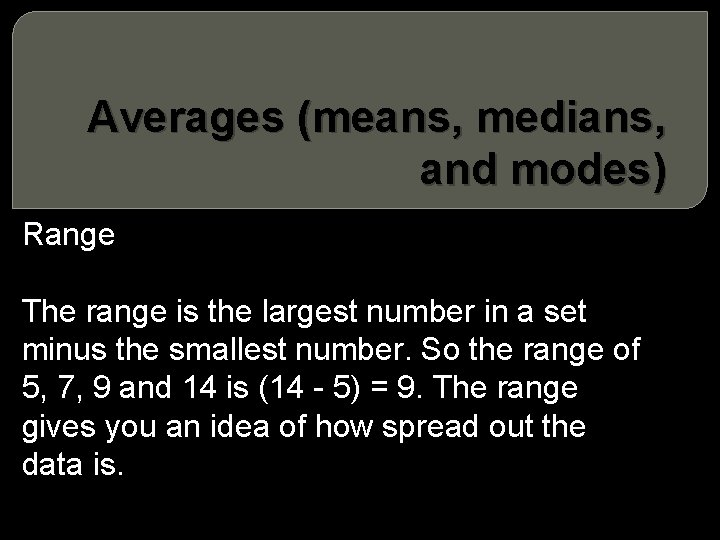Averages (means, medians, and modes) Range The range is the largest number in a set minus the smallest number. So the range of 5, 7, 9 and 14 is (14 - 5) = 9. The range gives you an idea of how spread out the data is.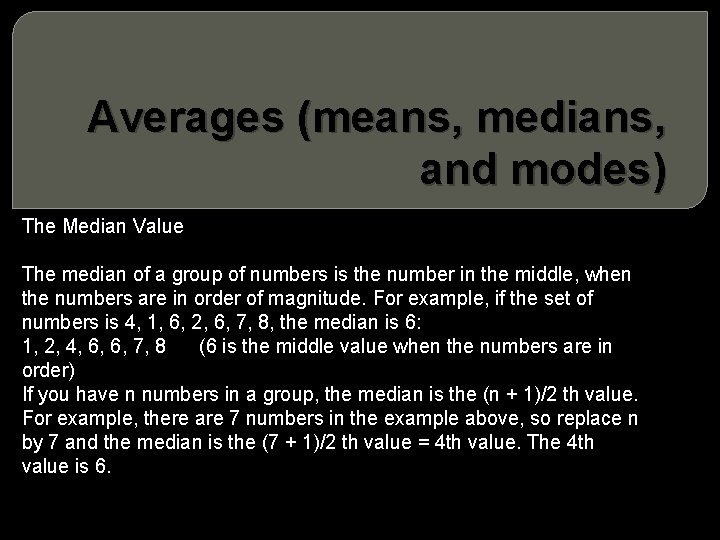Averages (means, medians, and modes) The Median Value The median of a group of numbers is the number in the middle, when the numbers are in order of magnitude. For example, if the set of numbers is 4, 1, 6, 2, 6, 7, 8, the median is 6: 1, 2, 4, 6, 6, 7, 8 (6 is the middle value when the numbers are in order) If you have n numbers in a group, the median is the (n + 1)/2 th value. For example, there are 7 numbers in the example above, so replace n by 7 and the median is the (7 + 1)/2 th value = 4 th value. The 4 th value is 6.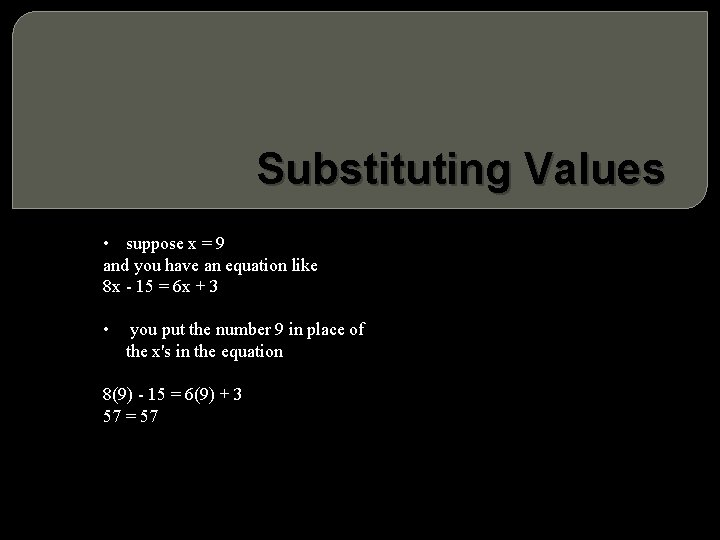Substituting Values • suppose x = 9 you have an equation like and - 15 = 6 x + 3 8 x • you put the number 9 in place of the x's in the equation 8(9) - 15 = 6(9) + 3 57 = 57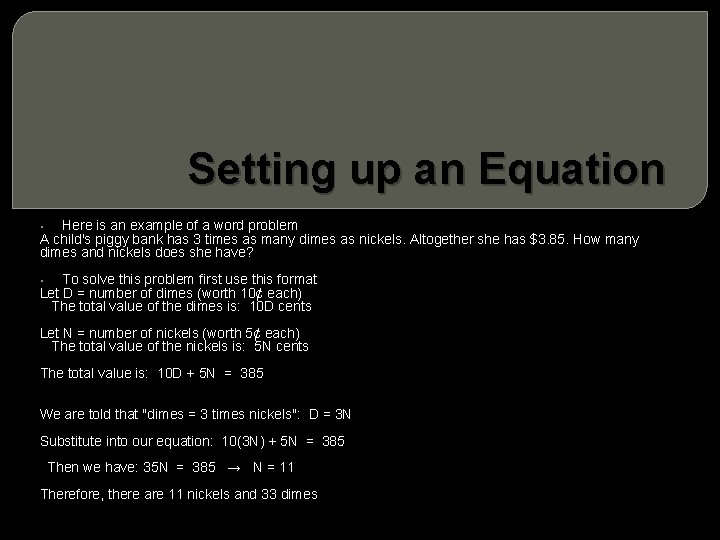Setting up an Equation Here is an example of a word problem A child's piggy bank has 3 times as many dimes as nickels. Altogether she has \$3. 85. How many dimes and nickels does she have? • To solve this problem first use this format Let D = number of dimes (worth 10¢ each) The total value of the dimes is: 10 D cents • Let N = number of nickels (worth 5¢ each) The total value of the nickels is: 5 N cents The total value is: 10 D + 5 N = 385 We are told that "dimes = 3 times nickels": D = 3 N Substitute into our equation: 10(3 N) + 5 N = 385 Then we have: 35 N = 385 → N = 11 Therefore, there are 11 nickels and 33 dimesFactoring Polynomials An example would be Factor 3 x-12 First identify what the two terms have in common Both terms are divisible by 3. When I divided the "3" out of the "3 x", I was left with only the "x" 3 x – 12 = 3(x ) When I divided the "3" out of the "– 12", I left a "– 4" behind, so I'll put that in the parentheses, too: 3 x – 12 = 3(x – 4) • This is my final answer: 3(x – 4)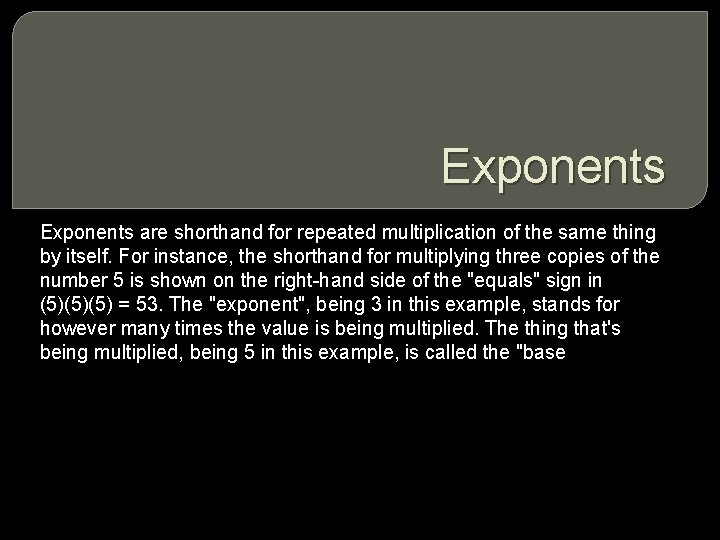Exponents are shorthand for repeated multiplication of the same thing by itself. For instance, the shorthand for multiplying three copies of the number 5 is shown on the right-hand side of the "equals" sign in (5)(5)(5) = 53. The "exponent", being 3 in this example, stands for however many times the value is being multiplied. The thing that's being multiplied, being 5 in this example, is called the "baseRadicals A "radical" equation is an equation in which at least one variable expression is stuck inside a radical, usually a square root Example For instance, this is a radical equation: There a couple of issues that frequently arise when solving radical equations. The first is that you must square sides, not terms. Here is a classic example of why this is so: I start with a true equation and then square both sides: 3 + 4 = 7 (3 + 4)2 = 72 49 = 49 . . . but if I square the terms on the left-hand side: 3 + 4 = 7 32 + 42 "=" 72 9 + 16 "=" 49 25 "=" 49. . . . Oops!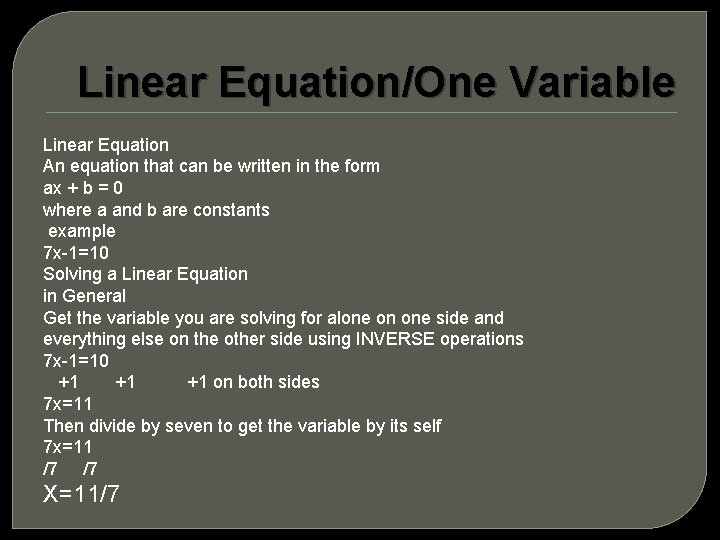Linear Equation/One Variable Linear Equation An equation that can be written in the form ax + b = 0 where a and b are constants example 7 x-1=10 Solving a Linear Equation in General Get the variable you are solving for alone on one side and everything else on the other side using INVERSE operations 7 x-1=10 +1 +1 +1 on both sides 7 x=11 Then divide by seven to get the variable by its self 7 x=11 /7 /7 X=11/7Sources www. purplemath. com www. mathgoodies. com www. mathsrevision. netFor Additional Help everything: http: //www. gamequarium. org/dir/Gamequarium/Math/ www. khanacademy. org polynomials: http: //www. coolmath. com/algebra-practice-polynomials. html algebra: http: //www. math. com/practice/Algebra. html pre-algebra: http: //www. math. com/practice/Pre. Algebra. html COMPASS specific: http: //www. act. org/compass/sample/math. html